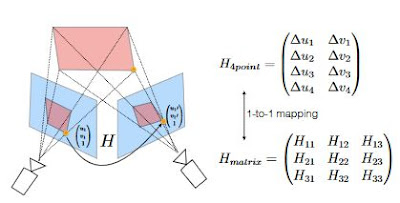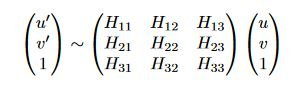## Tuesday, 10 October 2017

### Deep learning 11-Modern way to estimate homography matrix(by light weight cnn)

Today I want to introduce a modern way to estimate relative homography between a pair of images. It is a solution introduced by the paper titled Deep Image Homography Estimation.

## Introduction

Q : What is a homography matrix?

A : Homography matrix is a 3x3 transformation matrix that maps the points in one image to the corresponding points in another image.

Q : What are the use of homography matrix?

A : There are many applications depend on the homography matrix, a few of them are image stitching, camera calibration, augmented reality.

Q : How to calculate a homography matrix between two images?

A : Traditional solution are based on two steps, corner estimation and robust homography estimation. In corner detection step, You need at least  4 points correspondences between the two images, usually we would find out these points by matching features like AKAZE, SIFT, SURF.  Generally, the features found by those algorithms are over complete, we would prune out the outliers(ex : by RANSAC) after corner estimation. If you are interesting about the whole process describe by c++, take a look at this project.

Q : Traditional solution require heavy computation, do we have another way to obtain homography matrix between two images?

A : This is the question the paper want to answer, instead of design the features by hand, this paper design an algorithm to learn the homography between two images. The biggest selling point of the paper is they turn the homography estimation problem into a machine learning problem.

## HomographyNet

This paper use VGG style CNN to measure the homography matrix between two images, they call it HomographyNet. This model is trained in an end to end fashion, quite simple and neat.Fig00

HomographyNet come with two versions, classification and regression. Regression network produces eight real value numbers and use L2 loss as the final layer. Classification network use softmax as the final layer and quantize every real values into 21bins. First version has better accuracy, while average accuracy of second version is much worse than first version, it can produce confidences.Fig01

## 4-Point Homography Parameterization

Instead of using a 3x3 homography matrix as the label(ground truth), this paper use 4-point parameterization as label.

Q : What is 4-point parameterization?

A : 4-point parameterization store the different of 4 corresponding points between two images, Fig03 and Fig04 explain it well.Fig03Fig04
Q : Why do they use 4-point parameterization but not 3x3 matrix?

A : Because the 3x3 homography is very difficult to train, the problem is the 3x3 matrix mixing
rotation and translation together, the paper explain why.

The submatrix [H11, H12; H21, H22] represents the rotational terms in the homography., while the vector [H13, H23] is the translational offset. Balancing the rotational and translational terms as part of an optimization problem is difficult.Fig05

## Data Generation

Q : Training deep convolution neural networks from scratch requires a large amount of data, where could we obtain the data?

A : The paper invent a smart solution to generate nearly unlimited number of labeled training examples. Fig05 summarize the whole processFig06

## Results

Results of my implementation is outperform the paper, average loss of mine is 2.58, while the paper is 9.2. Largest loss of my model is 19.53. Performance of my model are better than the paper more than 3.5 times(9.2/2.58 = 3.57).  What makes the performance improve so much?A few of reasons I could think of are

1. I change the network architectures from vgg like to squeezeNet1.1 like.
2. I do not apply any data augmentation, maybe blurring or occlusion cause the model harder to train.
3. The paper use data augmentation to generate 500000 data for training, but I use 500032 images from imagenet as my training set. I guess this potentially increase variety of the data, the end result is network become easier to train and more robust(but they may not work well for blur or occlusion).

Following are some of the results, the region estimated by the model(red rectangle) is very close to the real regions(blue rectangle).Fig07

## Final thoughts

The results looks great, but this paper do not answer two important questions.

1. The paper only test on synthesis images, do they work on real world images?
2. How should I use the trained model to predict a homography matrix?

I would like to know the answer, if anyone find out, please leave me a message.

## Codes and model

As usual, I place my codes at github, model at mega.